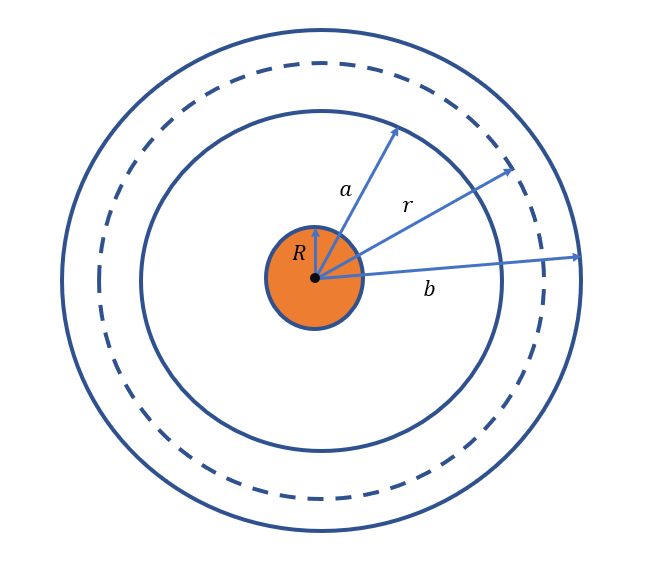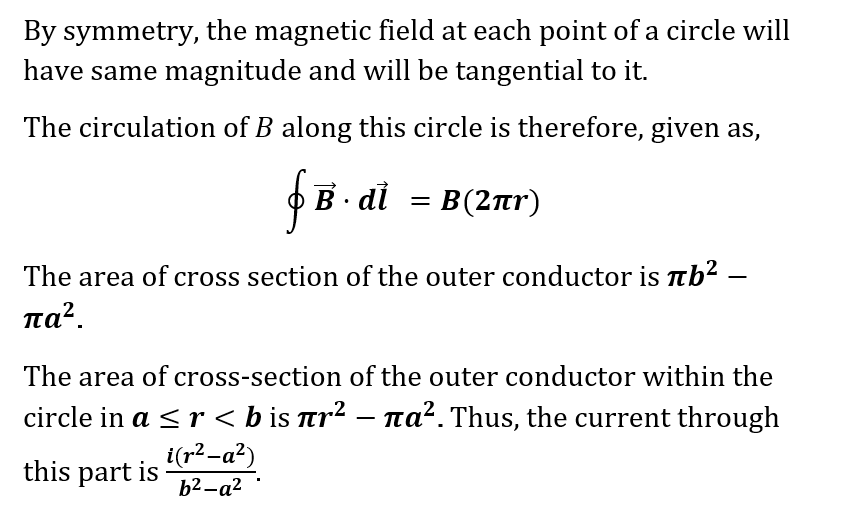# Consider a cylindrical coaxial cable. The center conductor is solid with radius of R, while the outer conductor is hollow with inner radius a and outer radius b. Both conductors have the same total current I (of uniform current density), moving in the opposite direction of each other. Find the magnitude of the magnetic field as a function of r (where r = 0  at the central axis) in the interval a is less than or equal to r which is less than b.I am unsure of how to start this problem and set up the correct equation.

Question
280 views

Consider a cylindrical coaxial cable. The center conductor is solid with radius of R, while the outer conductor is hollow with inner radius a and outer radius b. Both conductors have the same total current I (of uniform current density), moving in the opposite direction of each other. Find the magnitude of the magnetic field as a function of r (where r = 0  at the central axis) in the interval a is less than or equal to r which is less than b.

I am unsure of how to start this problem and set up the correct equation.

check_circle

Figure...

### Want to see the full answer?

See Solution

#### Want to see this answer and more?

Solutions are written by subject experts who are available 24/7. Questions are typically answered within 1 hour.*

See Solution
*Response times may vary by subject and question.
Tagged in